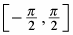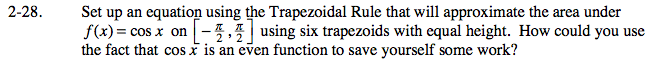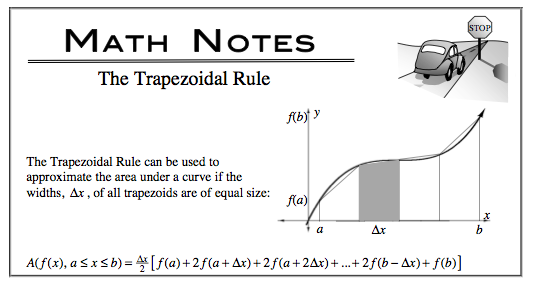### Home > CALC > Chapter 2 > Lesson 2.1.2 > Problem2-28

2-28.

Set up an equation using the Trapezoidal Rule that will approximate the area under f(x) = cos x onusing six trapezoids with equal height. How could you use the fact that cos x is an even function to save yourself some work? Homework Help ✎Notice the symmetry about the y-axis. If we evaluate the trapezoidal sum on one side of the y-axis, can we determine the trapezoidal sum on the other side of the y-axis?